### Home > CALC > Chapter 5 > Lesson 5.4.1 > Problem5-142

5-142.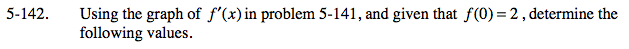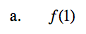The area under the curve between 0 and 1 represents displacement (or accumulated area).
However, it is given that f(0) = 2, which means f(x) has a starting value of y = 2.

$f(0)+\int_{0}^{1}f'(x)dx=\underline{ \ \ \ \ \ \ \ \ \ \ \ }$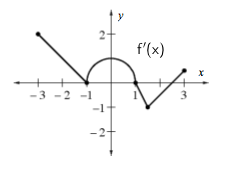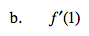Notice that the graph is of f '(x).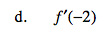Refer to hint in part (b).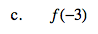Refer to hint in part (a).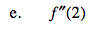f ''(x) represents the slope of f '(x) and you are looking at the f '(x) graph.